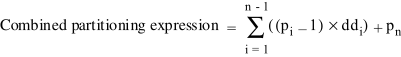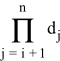# 17.10 - Combined Partitioning Expressions - Advanced SQL Engine - Teradata Database

## Teradata Vantage™ - SQL Data Definition Language Detailed Topics

Product
Release Number
17.10
Published
July 2021
Language
English (United States)
Last Update
2021-07-27
dita:mapPath
imq1591724555718.ditamap
dita:ditavalPath
imq1591724555718.ditaval
dita:id
jbg1472252759029
This topic describes what combined partitioning expressions are and how Vantage uses them.
• Vantage derives a combined partitioning expression from the partitioning expressions defined for each individual row partitioning level and a column partition number of 1 for the column-partitioning level.

For n partitioning levels, where n is the number of partitioning levels defined, the combined partitioning expression is defined as follows.where:

Equation element Description
p i Row partitioning expression at level i, numbering from left to right, or 1 for a column partitioning level.
dd i
Constant value equal to the following product.d j Maximum partition number defined at level j.

The parentheses delimiting the summation are not included in the combined partitioning expression. For example, this summation expands for 1-, 2-, 3-, 4-, and 5-level partitioning as the following combined partitioning expressions, respectively, where pi and ddi are defined as they were in the preceding definition for a combined partitioning expression.

Partitioning Level Expanded Summation
1 p 1
2 (p1-1)*dd1+p2
3 (p1-1)*dd1+(p2-1)*dd2+p3
4 (p1-1)*dd1+(p2-1)*dd2+(p3-1)*dd3+p4
5 (p1-1)*dd1+(p2-1)*dd2+(p3-1)*dd3+(p4-1)*dd4+p5

The combined partitioning expression reduces to p1 for single-level row partitioning, so there is no actual change in the usage rules for this case.

For column partitioning, the combined partition number for a specific column partition value of a table row can be derived from the combined partition number for this table row as follows:

combined_part_number-specific_col_part_value_of_row = cpn + (c - 1) * dd i

where:

Equation Element Description
combined_part_number-specific_col_part_value_of_row Combined partition number for a specific column partition value of a table row.
cpn Combined partition for the row.
c Column partition number for this column partition value within the row.
dd i Constant value equal to 1.

This is the same as computing the combined partitioning expression for a row-partitioned table row except instead of using 1 for the column-partitioning level, this equation uses the column partition number corresponding to the specific column partition value of the table row.

While column partitioning can be defined at any level, it is recommended in most cases to put the column-partitioning level first before any row partitioning. Some considerations that might lead to assigning the column partitioning to a lower level in the partitioning hierarchy are potential improvements for cylinder migration and temperature-based block compression effectiveness for hot and cold data.

The combined partitioning expression defines how rows are ultimately partitioned on each AMP. The result of the combined partitioning expression for specific values of the partitioning column is referred to as the combined partition number.

• With multilevel partitioning, the number of partitions defined by the combined partitioning expression is likely to be large. If there is a large number of populated partitions, performance of primary index access, joins, and aggregations on the primary index might be degraded. Therefore, multilevel partitioning may not be an appropriate choice when these operations occur frequently without significant simultaneous row partition elimination. However, a large number of row partitions for the combined partitioning expression allows for finer row partition elimination. See Determining the Partitioning Granularity.